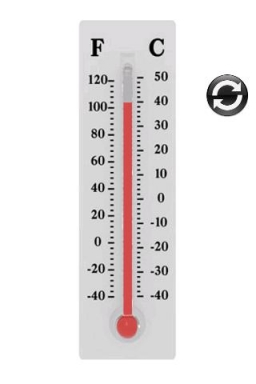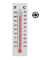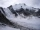# At noon 2

At noon, the temperature was 4 degrees fahrenheit. At 6pm, it was 10 degrees colder. What was the temperature at 6pm?

t2 =  -6 °F

### Step-by-step explanation:Did you find an error or inaccuracy? Feel free to write us. Thank you!## Related math problems and questions:

• Temperature change 3At 2 PM, The temperature was 76 degrees Fahrenheit. At 8 PM, the temperature was 58 degrees Fahrenheit. What was the change in temperature?
• Temperature variationsToday's temperature was 80 degrees, and then the temperature dropped 10 degrees. Then it dropped 15 degrees again, then the next day, the temperature went up 2 degrees. What would the temperature be?
• Temperature up and dowmAt 6 AM, the temperature is 19.4°C. Between 6 AM and noon, the temperature rises 3.8°C. Between noon and 6 PM, the temperature falls 2.5°C. What is the temperature at 6 PM?
• The temperature 16The temperature at 6 am was 21 degrees Fahrenheit by noon, and the temperature dropped 32 degrees Fahrenheit. What was the temperature at noon?
• NoontimeIn the morning temperature was 110°F. By noontime, it has gone up by 15°F. What was the noon temperature? (Give your answer in °F)At a weather centre, the temperature at midnight was -2 degree Celsius and by noon it had raised 4 degree Celsius. What is the new temperature?
• At noonAt noon today it was 6°C outside, but it has increased 4°C since then. What is the temperature outside now?
• OpheliaOphelia recorded the temperature of a cold store every two hours. 1. At 6 am, it was -4°C, and at 8 am, it was -1°C. By how much did the temperature rise? 2. The temperature went up by 5°C in the next two hours. .What was the temperature at 10 am?
• The temperature 9The temperature at 9 AM is 2 degrees. The temperature rises 3 more degrees by noon. Which expression describes the temperature at noon? Calculate the new temperature value.
• Temperature increaseIf the temperature at 9:am is 50 degrees. What is the temperature at 5:00pm if the temperature increases 4 degrees Fahrenheit each hour?
• The temperature 6The temperature was 47°F on Thursday and 60°F on Friday. How much did the temperature rise?
• Change in temperatureStarting temperature is 21°C, the highest temperature is 32°C. What is the change in temperature?
• The temperature 3The temperature is -12 degrees Fahrenheit. During the following 5 hours, the temperature decrease by 23 degrees Fahrenheit. What is the weather at 11 pm?
• The temperature 5The temperature at 4pm was 53° Fahrenheit the temperature dropped 3° each hour from 4pm to 9pm which equation below accurately represents the temperature at 9pm
• The temperature 4The temperature was very cold, then it doubled, then it dropped 10 degrees, and then it increased by 40 degrees. The temperature is now 16 degrees. What was the starting temperature?
• The outdoorThe outdoor temperature was 60 degrees Fahrenheit at midnight. The temperature went down 3 degrees each hour for the next 4 hours. What was the temperature at 4 A. M. ?
• Temperature rise and fallOn Friday, the temperature was 82°F. The temperature changed by –2°F on Saturday, and then it changed by 5°F on Sunday. What was the temperature on Sunday? How did the temperature change? Note we consider the mathematical problem formulated in this way to